Next: Degeneracy Up: Fundamental Concepts Previous: Measurements

# Expectation Values

Consider an ensemble of microscopic systems prepared in the same (normalized) initial state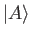. Suppose that a measurement of the observable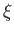is made on each system. We know that each measurement yields the value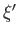with probability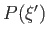. What is the mean value of the measurement? This quantity, which is generally referred to as the expectation value of, is given by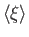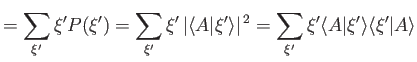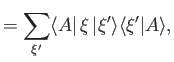(1.61)

which reduces to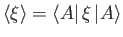(1.62)

with the aid of Equation (1.57).

Consider the identity operator, 1. All states are eigenstates of this operator with the eigenvalue unity. Thus, the expectation value of this operator is always unity: that is,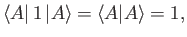(1.63)

for all. Note that it is only possible to normalize a given state ket, such that Equation (1.63) is satisfied, because of the more general property (1.22) of the norm. This property depends on the previously adopted correspondence, (1.11) and (1.16), between the elements of a ket space and those of its dual bra space.Next: Degeneracy Up: Fundamental Concepts Previous: Measurements
Richard Fitzpatrick 2016-01-22